# Angular and orbital speed at perihelion

pobro44
Homework Statement:
I want to derive how are angular and orbital speeds related in perihelion of eliptical orbit
Relevant Equations:
Angular momentum of reduced body in polar coordinates
Hello to all good people of physics forums. I just wanted to ask, whether the angular and linear (orbital) speed in perihelion of eliptical orbit are related the same way as in circular orbit (v = rw). If we take a look at the angular momentum (in polar coordinates) of reduced body moving in eliptical orbit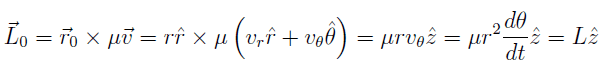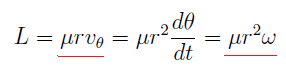if we equate the underlined factors and solve for v_theta, we get v_theta = rw. As I understand v_theta is a component of velocity perpendicular to position vector, and generaly is not equal to orbital velocity, but it is in perihelion/aphelion.

Is this reasoning correct? Thank you for taking the time to read and respond :).

Last edited:

Homework Helper
Gold Member
It's simpler than that. At perihelion/aphelion the radial component of the velocity vector is zero by definition. So the velocity is perpendicular to the radial direction at these two points.

•pobro44
pobro44
Thank you kuruman, I also derived a general expression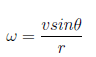where v is the orbital (tangential) velocity, and theta is the angle between velocity and position vector.

I derived it by using the magnitude of angular momentum of reduced body (one body problem)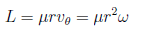and equating that expression with general one for angular momentum L = μrvsinθ and solving for ω. In polar coordinates, vsinθ is actually speed in theta hat direction, and in perihelion/aphelion theta is 90 degrees and angular speed becomes ω = v/r. However, my professor claimed this was wrong, and if I remember correctly, that I can't equate those two expression for angular momentum, but I can't figure out why.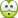Last edited: